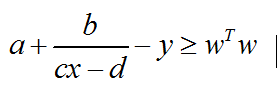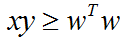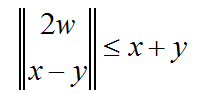# Does this constraint is SOC representable？

Hi, the constraint iswhere x,y are variables, a b c and d are real numbers. I know if the function has the follow structure:It is SOC representable and can be expressed asSo, I want to know, can the first constraint be expressed as a SOC constraint? If it can not, how can it be transformed so that it can be solved by CVX？

Thanks.

How have you proven it is convex (that determination is your responsibility)? Are w, x, and y your variables? What are the possible values of b and c*x - d?

Anyhow, if b <= 0, you can enter
`a + b*inv_pos(c*x-d) - y >= w'*w`
which is convex, although I leave it to your determination whether it correctly models what you want.

It is your responsibility to sort through all that and to determine what is convex, to include what are allowable values of parameters and constraints on all variables. When you have some formulation that is convex, you can seek assistance in how to implement it in CVX. I have shown how to represent one possible model formulation in CVX.